# HSSlive: Plus One & Plus Two Notes & Solutions for Kerala State Board

## AP Board Class 7 Maths Chapter 13 Area and Perimeter Ex 3 Textbook Solutions PDF: Download Andhra Pradesh Board STD 7th Maths Chapter 13 Area and Perimeter Ex 3 Book AnswersAP Board Class 7 Maths Chapter 13 Area and Perimeter Ex 3 Textbook Solutions PDF: Download Andhra Pradesh Board STD 7th Maths Chapter 13 Area and Perimeter Ex 3 Book Answers

## Andhra Pradesh State Board Class 7th Maths Chapter 13 Area and Perimeter Ex 3 Books Solutions

 Board AP Board Materials Textbook Solutions/Guide Format DOC/PDF Class 7th Subject Maths Chapters Maths Chapter 13 Area and Perimeter Ex 3 Provider Hsslive

2. Click on the Andhra Pradesh Board Class 7th Maths Chapter 13 Area and Perimeter Ex 3 Answers.
3. Look for your Andhra Pradesh Board STD 7th Maths Chapter 13 Area and Perimeter Ex 3 Textbooks PDF.
4. Now download or read the Andhra Pradesh Board Class 7th Maths Chapter 13 Area and Perimeter Ex 3 Textbook Solutions for PDF Free.

## AP Board Class 7th Maths Chapter 13 Area and Perimeter Ex 3 Textbooks Solutions with Answer PDF Download

Find below the list of all AP Board Class 7th Maths Chapter 13 Area and Perimeter Ex 3 Textbook Solutions for PDF’s for you to download and prepare for the upcoming exams:

Question 1.
Find the area of each of the following triangles.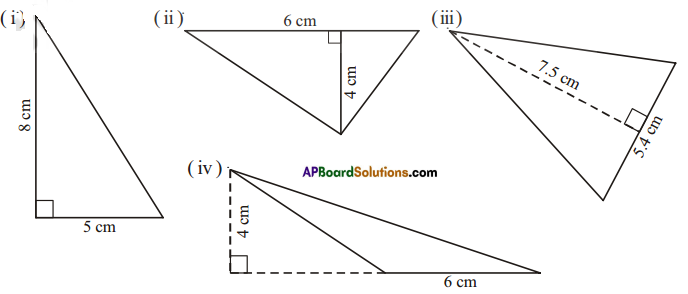Solution:
î) Area = 12 = 12 x 5 x 8 = 20 cm2
ii) Area = 12 = 12 x 6 x 4 = 12 cm2
iii) Area = 12 = 12 x 5.4 x 7.5 = 20.25 cm2
iv)Area = 12 = 12 x 6 x 4 = 12 cm2

Question 2.
In ΔPQR, PQ = 4 cm, PR =8 cm and RT = 6cm. Find (i) the area of ΔPQR (ii) the length of QS.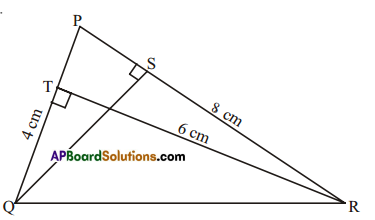Solution:
Given PQ 4cm, PR = 8cm, RT = 6cm
1) Area of ΔPQR = 12 × base × height = 12 × PQ × RT
= 12 × 4 × 6 = 12cm2

ii) Also area of ΔPQR = 12 × base x height = 12 × PR × QS
12 = 12 × 8 × QS
QR = 12×28 = 3cm

Question 3.
ΔABC is right-angled at A. AD is perpendicular to BC, AB =5 cm, BC = 13 cm and AC = 12 cm. Find the area of ΔABC. Also, find the length of AD.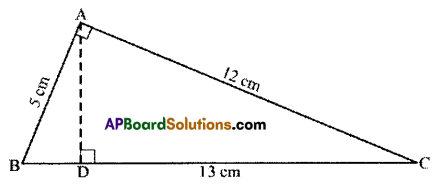Solution:
Given.
In ΔABC, ∠A = 90°
AB = 5cm; AC = 12cm; AD ⊥ BC; BC = 13cm.
Now area of ΔABC = 12 × base × height
= 12 x AB x AC = 12 × 5 × 12 = 30cm2
Also area of MBC = 12 × BC × AD
30 = 12 × 13 × AD
∴ 30×213=6013=4813 cm

Question 4.
ΔPQR is isosceles with PQ = PR = 7.5 cm and QR = 9 cm. The height PS from P to QR, is 6 cm. Find the area of ΔPQR. What will be the height from R to PQ i.e. RT?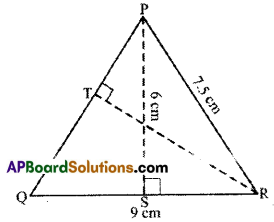Solution:
Given: PQ = PR = 7.5cm, QR = 9cm PS = 6cm
Area of ΔPQR = 12 bh = latex]\frac { 1 }{ 2 }[/latex] x 9 x 6 = 27cm2
Also area of ΔPQR = 12 × PQ × RT
27 = 12 × 7.5 × RT [PQ = PR]
∴ RT = 2×277.5=2×27×215=365 = 7.2 cm

Question 5.
ABCD rectangle with AB =8 cm, BC = 16 cm and AE = 4 cm. Find the area of ABCE. Is the area of ΔBEC equal to the sum of the area of ΔBAE and ΔCDE. Why?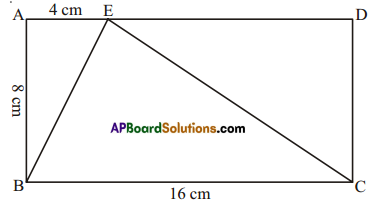Solution:
Given : In rectangle ABCD,
AB = 8cm, BC = 16 cm, AE = 4cm
Now Area of ΔBAE = 12 x base x height
= 12 × BC⎯⎯⎯⎯⎯⎯⎯⎯×AB⎯⎯⎯⎯⎯⎯⎯⎯
= 12 × 16 x 8 = 64cm2

Area of ΔCDE = 12 x base x height
= 12 × ED⎯⎯⎯⎯⎯⎯⎯⎯×DC⎯⎯⎯⎯⎯⎯⎯⎯
= 12 × 12 × 8 = 48cm2
(∵ ED⎯⎯⎯⎯⎯⎯⎯⎯ = AD – AE = 16 – 4 = 12cm and DC⎯⎯⎯⎯⎯⎯⎯⎯=AB⎯⎯⎯⎯⎯⎯⎯⎯)
Now ΔBAE + ΔCDE = 16 + 48 = 64 = ΔBCE.

Question 6.
Ramu says that the area of ΔPQR is, A = 12 × 7 × 5 cm2.
Gopi says that it is, A = 12 × 8 × 5 cm2. Who is correct’? Why?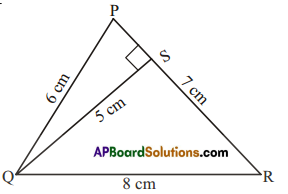Solution:
Ramu is correct.
Since area of a triangle = 12 × base × corresponding height
= 12 × 7 × 5cm2
But height 5cm is not corresponding to side &m.
∴ Gopi is not correct.

Question 7.
Find the base of a triangle whose area is 220 cm2 and height is 11cm.
Solution:
Given Area = 220 cm2, h = 11 cm
Area of a triangle = 12b.h
= 12 × b × 11 = 220cm2 (given)
b × 11 = 220 × 2
b = 220×211 = 40
∴ base of the triangle = 40 cm.

Question 8.
In a triangle the height is double the base and the area is 400 cm2. Find the length of the base and height.
Solution:
Area = 400 cm2
Let the base of the triangle be x.
height = 2x
Area of the triangle = 12b.h
12 × x × 2x = 400 (given)
x2 = 400
x = 400‾‾‾‾√ = 20
∴ base of the triangle = 20 cm
height = 2(base)= 2(20) = 40 cm.

Question 9.
The area of triangle is equal to the area of a rectangle whose length and breadth are 20 cm and 15 cm respectively. Calculate the height of the triangle if its base measures 30 cm.
Solution:
Given :Length of the rectangle = 20 cm
Breadth of the rectangle = 15 cm
Base of the triangle = 30 cm
Area of the rectangle = Area of the triangle
Length x breadth = 12 × base × height
20 × 15 = 12 × 30 × height
∴ height = 20×1515 = 20cm

Question 10.
In Figure ABCD find the area of the shaded region.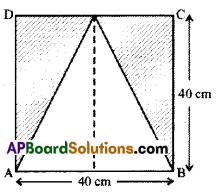Solution:
Given : Side of the square ABCD = 40cm
Area of the shaded rgion = (Area of the square) — (Area of the unshaded region)
= (side × side) – 12 × base × height
= 40 × 40 – 12 × 40 × 40
= 1600 – 800 = 800cm2

Question 11.
In Figure ABCD, find the area of the shaded region.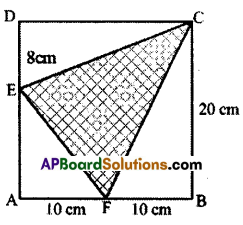Solution:
Given: Side of the square = 20 cm
= (Area of the square) – (Area of unshaded region)
= (Square ABCD) – (ΔEAF + ΔFBC + ΔEDC)
= side × side – (12AF × AE + 12 FB × BC + 12 DE × DC)
= 20 × 20 – (12 × 10 × 12 + 12 × 10 × 20 + 12 × 8 × 20)
= 400 – [60 + 100 + 80] = 400 – 240 = 160cm2

Question 12.
Find the area of a parallelogram PQRS, if PR =24 cm and QU = ST =8 cm.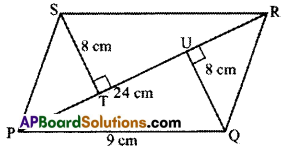Solution:
Given : In PQRS, PR = 24cm, ST = 8cm, QU =8cm
Area of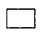PQRS = ΔPQR + ΔPRS
= 12 × 12base x height + x base x height
= 12 × 24 x 8 + 12 × 24 × 8 = 96 + 96 = 192 cm2

Question 13.
The base and height of the triangle are in the ratio 3:2 and its area is 108 cm2. Find its base
and height.
Solution:
Given : Area = 108 cm2
Let the base of the triangle be 3x and height of the triangle be 2x.
Area of the triangle = 12 × base × height = 12 x (3x) (2x) = 108 cm2 (given)
3x2 = 108
x2 = 108/3 = 36
x = 36‾‾‾√ = 6
∴ base 3x = 3(6) = 18 cm
height = 2x = 2(6) = 12cm

## Andhra Pradesh Board Class 7th Maths Chapter 13 Area and Perimeter Ex 3 Textbooks for Exam Preparations

Andhra Pradesh Board Class 7th Maths Chapter 13 Area and Perimeter Ex 3 Textbook Solutions can be of great help in your Andhra Pradesh Board Class 7th Maths Chapter 13 Area and Perimeter Ex 3 exam preparation. The AP Board STD 7th Maths Chapter 13 Area and Perimeter Ex 3 Textbooks study material, used with the English medium textbooks, can help you complete the entire Class 7th Maths Chapter 13 Area and Perimeter Ex 3 Books State Board syllabus with maximum efficiency.

## FAQs Regarding Andhra Pradesh Board Class 7th Maths Chapter 13 Area and Perimeter Ex 3 Textbook Solutions

#### Can we get a Andhra Pradesh State Board Book PDF for all Classes?

Yes you can get Andhra Pradesh Board Text Book PDF for all classes using the links provided in the above article.

## Important Terms

Andhra Pradesh Board Class 7th Maths Chapter 13 Area and Perimeter Ex 3, AP Board Class 7th Maths Chapter 13 Area and Perimeter Ex 3 Textbooks, Andhra Pradesh State Board Class 7th Maths Chapter 13 Area and Perimeter Ex 3, Andhra Pradesh State Board Class 7th Maths Chapter 13 Area and Perimeter Ex 3 Textbook solutions, AP Board Class 7th Maths Chapter 13 Area and Perimeter Ex 3 Textbooks Solutions, Andhra Pradesh Board STD 7th Maths Chapter 13 Area and Perimeter Ex 3, AP Board STD 7th Maths Chapter 13 Area and Perimeter Ex 3 Textbooks, Andhra Pradesh State Board STD 7th Maths Chapter 13 Area and Perimeter Ex 3, Andhra Pradesh State Board STD 7th Maths Chapter 13 Area and Perimeter Ex 3 Textbook solutions, AP Board STD 7th Maths Chapter 13 Area and Perimeter Ex 3 Textbooks Solutions,
Share: